# A useful formula for solenoid-inductor design

Home - Techniek - Electronica - Radiotechniek - Radio amateur bladen - QST - A useful formula for solenoid-inductor design

The design of inductors for radio work is usually straightforward, thanks to the work of Nagaoka and Wheeler and others who have developed accurate inductance formulas for almost every conceivable wire configuration. Most often the inductor is to be a solenoid of dimensions mechanically suited to its application. The mean diameter and the approximate winding length are known, and the problem becomes that of finding N, the number of turns necessary to resonate at f megacycles, when in circuit with C micromicrofarads. The inductance value for the winding, usually determined in the design, often has no immediate significance in itself.

A convenient formula is derived by substiting resonant-circuit parameters for L in eeler's solenoid-inductance formula and then g for N, the number of turns required, in of winding dimensions, frequency, and uit capacitance: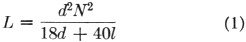where:
d = mean diameter, inches
l = winding length, inches
N = turns
L = inductance in microhenries

Solving for N,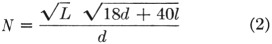The formula for frequency in terms of inductee and capacitance is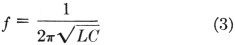Solving for L,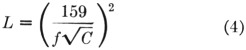where
f = frequency in Mc.
C = capacitance in pF.

Substituting in (2),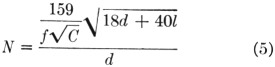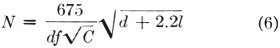To avoid decimal difficulties in calculation, C is not placed in the denominator of the radical form-factor term.

### Example

In illustration, let us design a solenoid to be wound on a 1-inch form in 1% inches winding space that will resonate at 7 Mc. with 100 pofd. of total capacitance.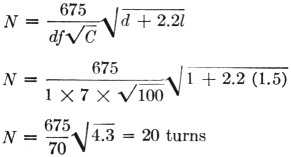If a solenoid is to be wound at a winding pitch of P turns per inch, N/P might be substituted for l in this formula and a solution again found for N, but unfortunately the expression and its calculation become lengthy. It is more expedient to arrive at N by assigning an estimated value to l in the formula, finding N by a rough calculation and then redetermining 1 from N so obtained and the stipulated winding pitch.

Wheeler's formula and this modification of it are accurate for all inductor form factors usually encountered in solenoid design. Some small error may be introduced in estimating distributed circuit capacitances that will parallel the lumped capacitance of the resonant circuit.

James B. Ricks, W9TO.